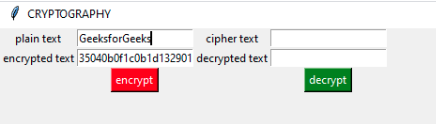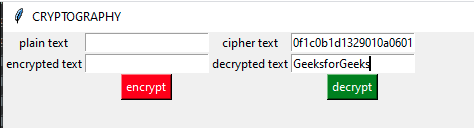# Cryptography GUI using python

Using cryptography techniques we can generate keys for a plain text which can not be predicted easily. We use Cryptography to ensure the safe and secure flow of data from one source to another without being accessed by a malicious user.

Prerequisites:

Language usedPython.
Tkinter – This module is used to make GUIs using python language. To know more about tkinter click here.
Basics of Cryptography – Cryptography is used for Secure Communication.

• Encryption – The process of encoding a message or information in such a way that only authorized parties can access it.
• Decryption – The process of taking encoded or encrypted text or other data and converting it back into text.
• #### Algorithm used

The `one-time pad` is a type of encryption which is unbreakable. A one-time pad will generate a key, this key is shared by both the user so it does encryption as well as decryption. The key used is generated randomly and this key is combined with the plain text in order to form the ciphertext. We can use different algorithms for the generation of the ciphertext such as `modular addition`,` modular XOR`, etc. Since the key generated every time is unique, it is impossible to break.

Examples:

In this example, we use a modular addition. Every letter of the message has it’s numerical value associated with it. This numerical value is mapped with the corresponding letter of the key and ciphertext is generated by doing modular addition operation. if the value exceeds 26, the result will be the mod of the value with 26. Here ‘GEEKS’ acts as a plain message and ‘DFSTL’ acts as the one-time pad key.

```      G     E      E       K      S      message
6 (G)   4 (E)  4 (E)   10 (K)  18 (S) message
+  3 (D)   5 (F)  18 (S)  19 (T)  11 (L) key
=  9       9      22      29      29     message + key
=  9 (J)   9 (J)  22 (W)  3 (D)   3 (D) (message + key) mod 26
J       J       W      D       D  ? ciphertext
```

Since we used modular addition for the generation of the ciphertext. In order to get back the original message we have to perform modular subtraction. If the value comes out to be negative we will add 26 to the value, the resultant numerical value will result in the generation of the original message.

```       J       J       W       D       D  ciphertext
9 (J)   9 (J)   22 (W)  3 (D)   3 (D) ciphertext
-   3 (D)   5 (F)  18 (S)  19 (T)  11 (L) key
=   6       4       4     -16      -8     ciphertext – key
=   6 (G)   4 (E)  4 (E)  10(K)    18 (S) ciphertext – key (mod 26)
G       E       E      K       S  ? message
```

Below is the implementation.

 `# python module for one-timepad ` `import` `onetimepad    ` `# python module to create GUI         ` `from` `tkinter ``import` `*`  ` `  `        `  `root ``=` `Tk() ` `root.title(``"CRYPTOGRAPHY"``) ` `root.geometry(``"800x600"``) ` ` `  `def` `encryptMessage():                       ` `    ``pt ``=` `e1.get() ` ` `  `    ``# inbuilt function to encrypt a message ` `    ``ct ``=` `onetimepad.encrypt(pt, ``'random'``) ` `    ``e2.insert(``0``, ct) ` ` `  `def` `decryptMessage():                      ` `    ``ct1 ``=` `e3.get() ` ` `  `    ``# inbuilt function to decrypt a message ` `    ``pt1 ``=` `onetimepad.decrypt(ct1, ``'random'``) ` `    ``e4.insert(``0``, pt1) ` `     `  `# creating labels and positioning them on the grid ` `label1 ``=` `Label(root, text ``=``'plain text'``)                ` `label1.grid(row ``=` `10``, column ``=` `1``) ` `label2 ``=` `Label(root, text ``=``'encrypted text'``) ` `label2.grid(row ``=` `11``, column ``=` `1``) ` `l3 ``=` `Label(root, text ``=``"cipher text"``) ` `l3.grid(row ``=` `10``, column ``=` `10``) ` `l4 ``=` `Label(root, text ``=``"decrypted text"``) ` `l4.grid(row ``=` `11``, column ``=` `10``) ` ` `  `# creating entries and positioning them on the grid ` `e1 ``=` `Entry(root) ` `e1.grid(row ``=` `10``, column ``=` `2``) ` `e2 ``=` `Entry(root) ` `e2.grid(row ``=` `11``, column ``=` `2``) ` `e3 ``=` `Entry(root) ` `e3.grid(row ``=` `10``, column ``=` `11``) ` `e4 ``=` `Entry(root) ` `e4.grid(row ``=` `11``, column ``=` `11``) ` ` `  `# creating encryption button to produce the output ` `ent ``=` `Button(root, text ``=` `"encrypt"``, bg ``=``"red"``, fg ``=``"white"``, command ``=` `encryptMessage) ` `ent.grid(row ``=` `13``, column ``=` `2``) ` ` `  `# creating decryption button to produce the output ` `b2 ``=` `Button(root, text ``=` `"decrypt"``, bg ``=``"green"``, fg ``=``"white"``, command ``=` `decryptMessage) ` `b2.grid(row ``=` `13``, column ``=` `11``) ` ` `  ` `  `root.mainloop() `

Output
For encryption:For decryption:Note: The default technique used by the module is not as same in the example given. We can apply different formulas for the generation of the ciphertext, however, the underlying principle remains the same.

Attention geek! Strengthen your foundations with the Python Programming Foundation Course and learn the basics.

To begin with, your interview preparations Enhance your Data Structures concepts with the Python DS Course.

My Personal Notes arrow_drop_upCheck out this Author's contributed articles.

If you like GeeksforGeeks and would like to contribute, you can also write an article using contribute.geeksforgeeks.org or mail your article to contribute@geeksforgeeks.org. See your article appearing on the GeeksforGeeks main page and help other Geeks.

Please Improve this article if you find anything incorrect by clicking on the "Improve Article" button below.

Article Tags :

Be the First to upvote.

Please write to us at contribute@geeksforgeeks.org to report any issue with the above content.